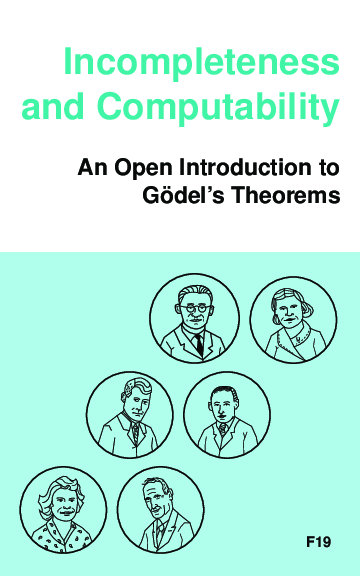# Incompleteness and Computability. An Open Introduction to Gödel’s Theorems

Textbook on Gödel’s incompleteness theorems and computability theory, developed for Calgary’s Logic III course, based on the Open Logic Project. Covers recursive function theory, arithmetization of syntax, the first and second incompleteness theorem, models of arithmetic, second-order logic, and the lambda calculus.